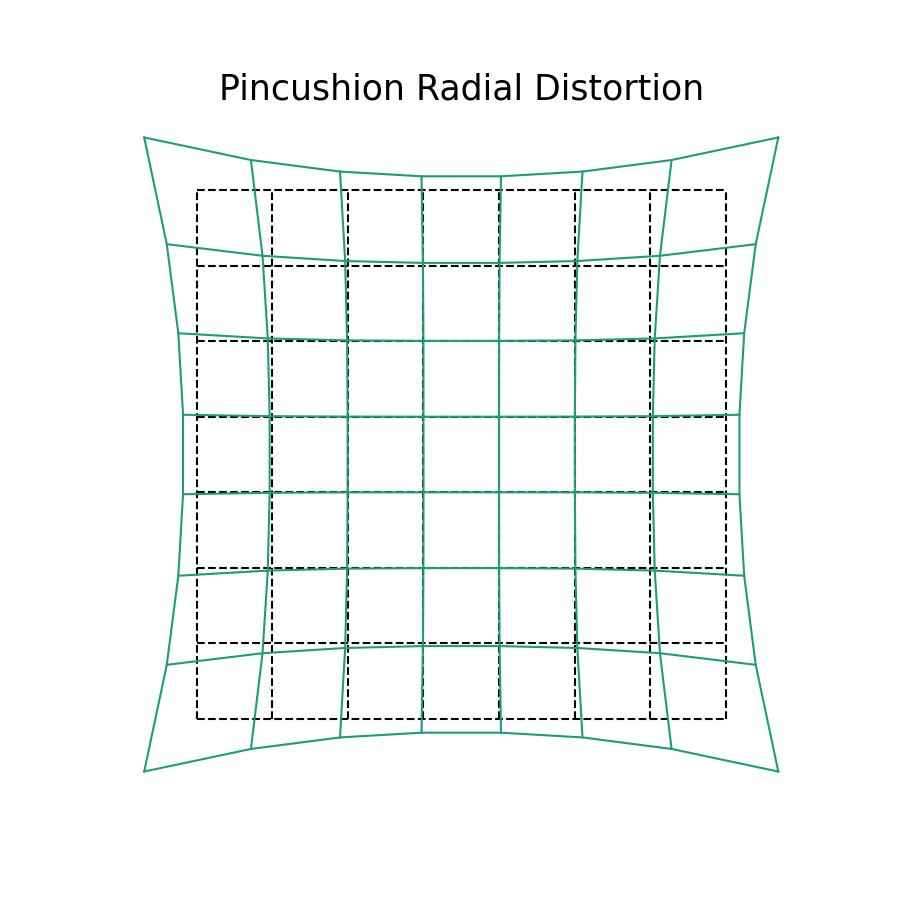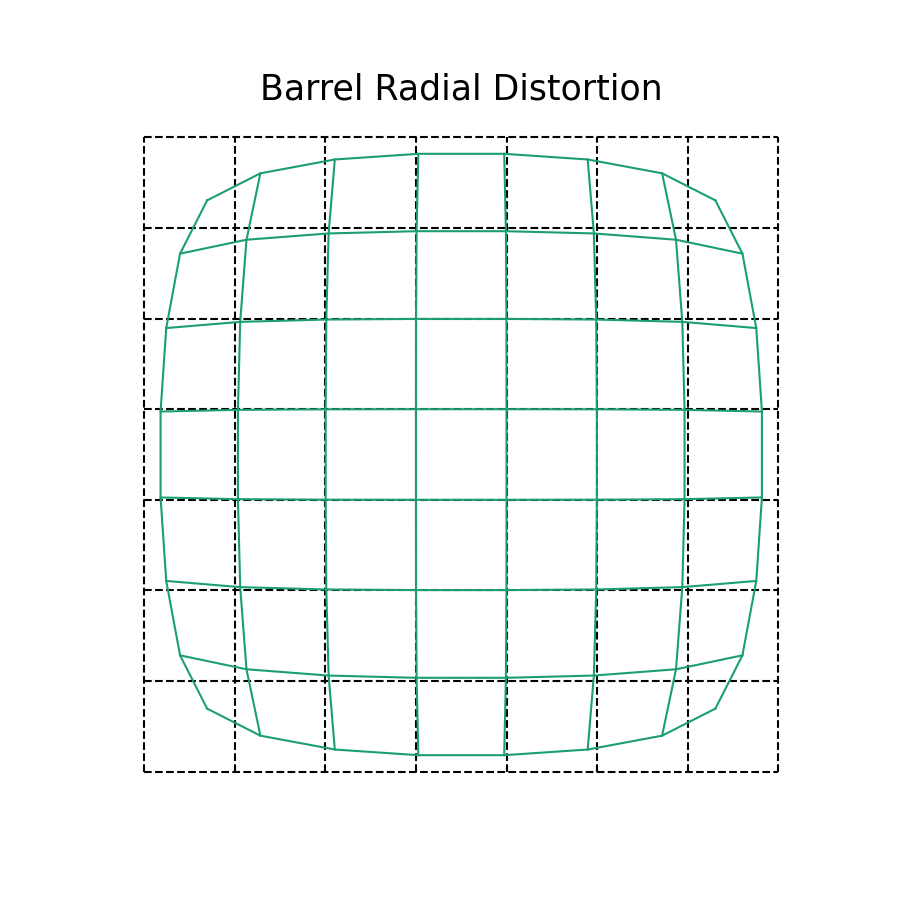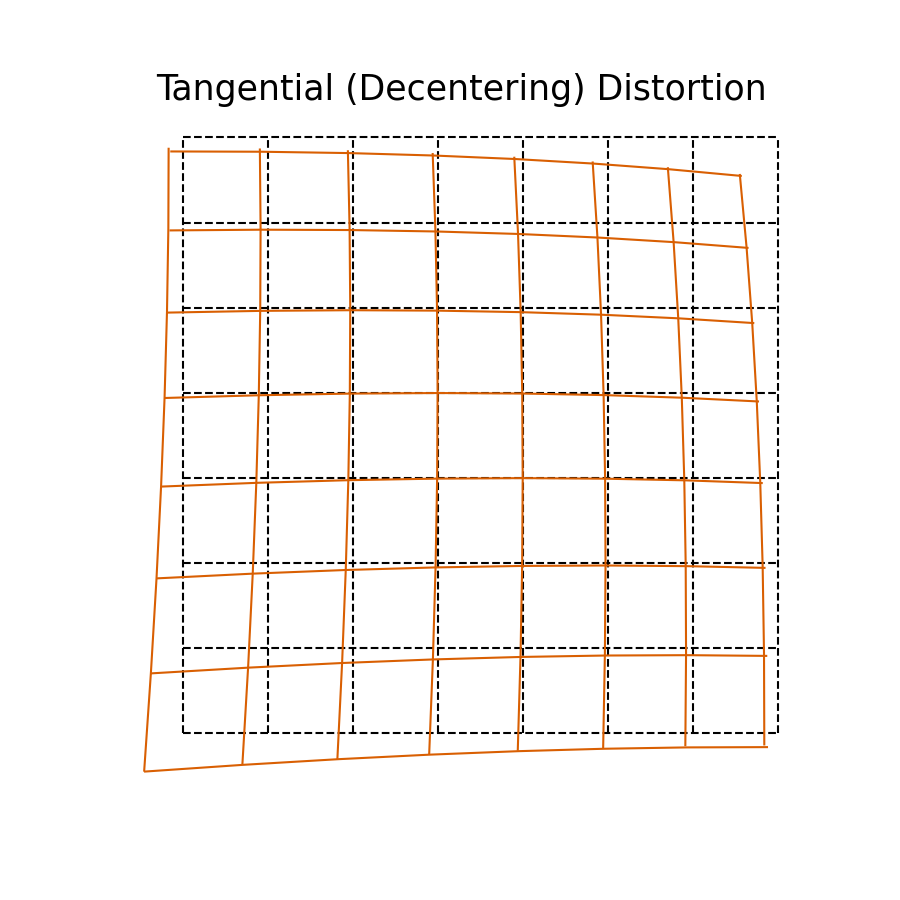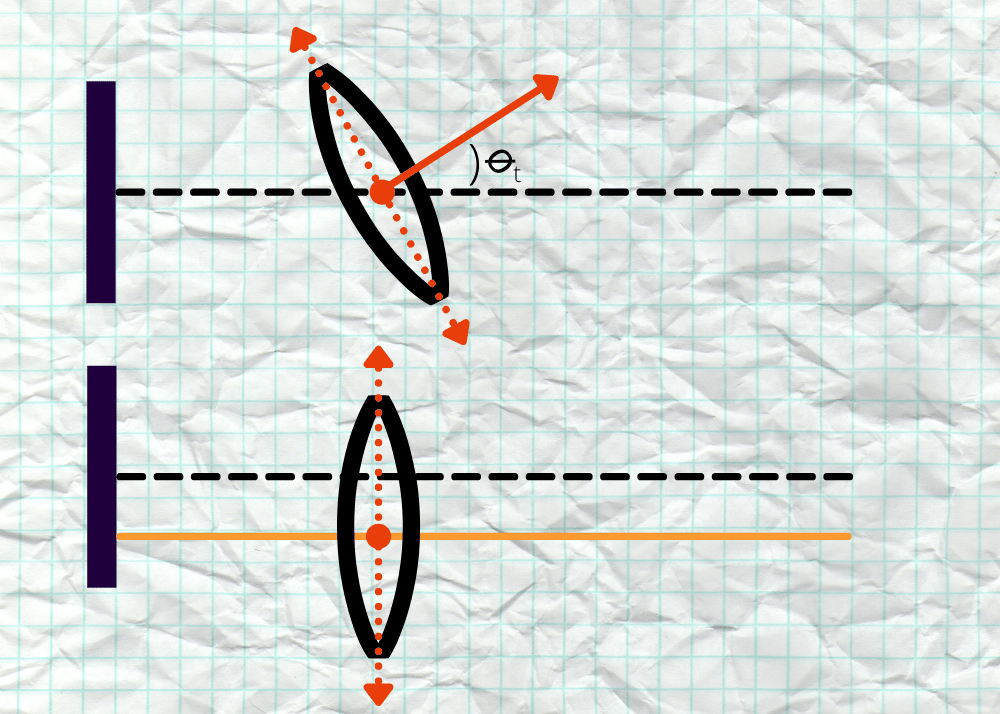< Back to Blog

# Camera Modeling: Exploring Distortion and Distortion Models, Part I

###### August 6, 2021
Tutorial

Previously, we covered some of the basics of camera modeling, and examined the pinhole projection model of a camera. There, we looked at some of the historical context behind how affinity in the image plane has been traditionally modeled, and why different models are preferable.

Today, we want to explore another part of the camera modeling process: modeling lens distortions. One assumption behind our pinhole-projection model is that light travels in straight rays, and does not bend. Of course, our camera's lens is not a perfect pinhole and light rays will bend and distort due to the lens' shape, or due to refraction. If you've already completed this post, head on over to Part II.

## What does lens distortion look like?

Before we get into the actual models themselves, it is good to first understand what lens distortion looks like. There are several distinct types of distortions that can occur due to the lens or imaging setup, each with unique profiles. In total, these are typically broken into the following categories:

3. Tangential or De-centering Distortion

Each of these is explored independently below. For each of these distortions, consider the base-case where there is no distortion:

In the above figure, we represent the image plane as a grid. As we discuss the different kinds of distortions, we'll demonstrate how this grid is warped and transformed. Likewise, for any point $$(x, y)$$ that we refer to throughout this text, we're referring to the coordinate frame of the image plane, centered (with origin) at the principal point. This makes the math somewhat easier to read, and doesn't require us to worry about column and row offsets $$c_x$$ and $$c_y$$ when trying to understand the math.

Symmetric radial distortions are what are typically imagined when discussing image distortion. Often, this type of distortion will be characterized depending on if it is positive (pincushion) or negative (barrel) distortion.Positive (pincushion) distortion. It is "positive" because the projected distance increases beyond the expected locations as one moves farther away from the centre of the image plane.Negative (barrel) distortion. It is "negative" because the projected distance decreases from the expected locations as one moves farther away from the centre of the image plane

While the two distortions might seem as if they are fundamentally different, they are in fact quite alike! The amount of distortion in the teal lines is greater in magnitude at the edges of our image plane, while being smaller in the middle. This is why the black and teal lines overlap near the centre, but soon diverge as distance increases.

Symmetric radial distortion is an after-effect of our lens not being a perfect pinhole. As light enters the lens outside of the perspective centre it bends towards the image plane. It might be easiest to think of symmetric radial distortion as if we were mapping the image plane to the convexity or concavity of the lens itself. In a perfect pinhole camera, there wouldn't be any distortion, because all light would pass through a single point!

This distortion is characterized as symmetric because it only models distortion as a function of distance from the centre of the image plane. The geometric effect of radial distortion is only in the radial direction, as characterized by $$\delta r$$ in the above figure.

Asymmetric radial distortions are radial distortion effects much like the above, but unlike symmetric radial distortion, asymmetric radial distortion characterizes distortion effects that are dependent both on the distance from the image centre as well as how far away the object being imaged is. Asymmetric radial effects are most pronounced in two scenarios:

1. Cameras with long focal lengths and very-short relative object distances. e.g. a very-near-field telephoto lens that is capturing many objects very close.
2. Observing objects through a medium of high-refraction, or differing refractive indices. e.g. two objects underwater where one is near and one is far away.

This type of distortion is typically tricky to visualize, as well as to quantify, because it is dependent on the environment. In most robotic and automated vehicle contexts, asymmetric radial distortion is not a great concern! Why? Well, the difference in distortions depends on the difference in distances between objects. This is usually because of some kind of refractive difference between two objects being imaged, or because the objects are out of focus of the camera (i.e. focal length is too large relative to the object distance).

Neither of the above two scenarios are typical; as such, asymmetric radial distortion is an important aspect of modeling the calibration in applications when these scenarios are encountered.

In most robotic contexts, the primary use for imaging and visual-odometry is done in relatively short ranges with cameras that have short focal lengths, and the primary medium for light to travel through is air. Since there doesn't tend to be big atmospheric variances between objects that are close, and since light is all traveling through the same medium, there isn't much of an asymmetric refractive effect to characterize or measure. As a result, this kind of radial distortion isn't common when calibrating cameras for these kinds of applications. If we can't measure it, we shouldn't try to model it!

💡 You may be left wondering what is meant by "close" when discussing "atmospheric variance between objects that are close." In short: it is all relative to the refractive index of the medium in which you are imaging. On the ground it is atypical to have atmospheric effects (we don't see pockets of ozone on the ground, as an example).

This is not generally true underwater, nor if you are performing some kind of aerial observation from the stratosphere or higher. Additionally, even in such scenarios, if all observed objects are roughly the same distance away then it doesn't matter since asymmetric radial distortion characterizes radial effects as a result of the extra distance light travels (and refracts) between two objects at different distances from the camera.

## Tangential (De-centering) Distortions

The last kind of distortions to characterize in a calibration are tangential effects, often as a result of de-centered lens assemblies. First, see the following figure for an (exaggerated) example of what these might look like:Tangential or de-centering distortion of the image plane. Notice that unlike radial distortion, the image plane is skewed, and the distance from center is less important. Note that here the effect is exaggerated, most cameras do not have tangential distortions to this degree.

Tangential distortion is sometimes also called de-centering distortion, because the primary cause is due to the lens assembly not being centered over and parallel to the image plane. The geometric effect from tangential distortion is not purely along the radial axis. Instead, as can be seen in the figure above, it can perform a rotation and skew of the image plane that depends on the radius from the image centre!Example of lens geometry as it impacts tangential distortion. In the top graphic, the lens is not parallel with the image plane, which produces tangential distortion. In the lower graphic, the lens is offset from the image plane. While we might normally model this with $$c_x$$ and $$c_y$$ for a single lens, if this is one lens offset among a collection of lenses, the final effect projects into our image as tangential or de-centering distortion.

In the above figure, one can see how the lens being either angled with respect to the orthogonal axis of the image plane, or shifted, would project an image into a different spot on the plane. In most cameras used for robotics or automated vehicle applications, tangential distortion will usually be significant enough to model, but is often an order of magnitude smaller than e.g. symmetric radial distortion.

## Compound distortions

Typically when we think of distortion, we try to break down the components into their constituent parts to aid our understanding. However, most lens systems in the real world will have what is often referred to as compound distortion. There's no tricks here, it's simply an aggregate effect of all the previous types of distortions in some combination. This kind of distortion is especially prevalent in cameras with compound lenses, or very complicated lens assemblies.

## Moving On From Part I to Part II

At this point, we've covered the different kinds of lens distortion types one can experience when working with camera-based systems. We'll pause here, and return with Part II, where we'll introduce common distortion models that can be used to correct these distortions, for more accurate calibration models and overall system performance. Read Part II now.

## Accelerating Perception

The Tangram Vision Platform lets perception teams develop and deploy faster.